Mobile QR Code1. (Department of Information & Communication Engineering, Hanbat National University, 125 Dongseodaero, Daejeon, 34158, Korea)

PUF, shrink generator, challenge-response-pair (CRP), configurable ring oscillator (CRO) PUF, strong PUF

## I. INTRODUCTION

The scope of applications of Field Programmable Gate Arrays (FPGAs) has broadened in recent years. FPGAs are used in embedded system designs due to their flexibility, reconfigurability, and low cost of design over their ASIC counterpart. Research areas such as big data and artificial intelligence are gravitating towards the use of FPGAs for their application development, by leveraging the powerful computing power and high flexibility that comes with it. For these reasons, mobile phone giant- iPhone - utilized ICE5LP4K low power lattice FPGAs in their iPhone 7 models . The CPUs in the data centers of Microsoft are being replaced with FPGAs to enhance machine learning applications .

The use of FPGAs in ubiquitous hardware is gaining popularity. As the presence of ubiquitous devices - known universally as the Internet of Things (IoT)- in our everyday life increases, more so are the various security challenges and issues they present. Some of the security issues faced because of using FPGAs include intellectual property theft where reverse engineering techniques are applied to extract IP or sensitive information from a chip, Integrated Chip (IC) tempering. This happens by way of inserting malicious codes such as kill switches and Hardware Trojans to grant unauthorized access of the host devices to adversaries and cloning (making a copy of an IC or IP) to resell . Building hardware devices that are immune'' to cloning or replication is a daunting task. Current technological advancement in IC design has made it complex to tell a genuine hardware device from a counterfeit or an imitated one.

A means by which some of these threats can be mitigated is through the use of PUFs. There have been numerous forms of PUFs proposed through research [4,5] ever since the concept was introduced by Pappau et al in . The PUFs that are suitable for Field Programmable Gate Arrays (FPGAs) can be categorized as either delay-based PUF or as memory-based PUF . Notable among the delay-based PUFs are the Ring Oscillator (RO) PUF  and the CRO-PUF [4,9]. Based on the propagation delay of the signals of each inverter and wire, the RO can exploit these delay variations that result in differences in oscillating frequency to its advantage. Generally, RO PUFs are much more suitable for implementation on FPGAs because they do not require a higher degree of symmetry to be successfully implemented as compared to the other PUFs. The RO PUFs and its variants have gained an increased usage in security for hardware IP protection, device authentication, and other areas of systems security. The main challenges of the RO PUF architectures are that, owing to the inadequate number of challenge-response pairs (CRPs), they fall under the Weak PUF category. Therefore, cannot be used for authentication purposes. Additionally, the outputs of this class of PUF’s are sensitive to changes in voltage, temperature, and other environmental conditions. This renders the responses of the RO PUFs highly unreliable. Given these limitations, contributions made to the PUF area research through this paper is a proposed CRO-PUF architecture that:

(1) Generates highly reliable responses using a limited number of multiplexers placed within the stages of the RO chain to make it reconfigurable and in effect, attenuate the environmental changes that influence the response.

(2) Improves upon the challenge-response pair set by the introduction of a Shrink Generator thereby increasing the scope of application of CRO-based PUFs.

The remainder of this paper is sectioned into the following parts to enhance the flow of discussions. In section II, the concept of realizing Strong PUFs from Weak PUFs is discussed. In III, the proposed Linear Feedback Shift register architecture is introduced. Section IV presents the proposed CRO-PUF architecture. Experimental setup, results, and discussions are presented in Section V. Finally, the conclusion and future work are discussed in Section VI.

## II. BUILDING STRONG PUFS FROM WEAK PUFS

PUFs are categorized into two important subtypes based on the number of CRP each architecture can generate. These subtypes are the Weak PUFs and Strong PUFs. The former, which is an alternative form of storing secrete keys, is characterized by having few or fixed CRPs per PUF. Strong PUFs, on the contrary, have a large or exponential CRP set, but they are not suitable for implementation on FPGAs. The number of CRP’s for the RO-PUF; a Weak PUF; with N-number of challenges is only 2, while the number of CRP’s for the Arbiter-PUF; a Strong PUF; with N challenges is $2^{\boldsymbol{N}}$. Although the size of the CRPs is exponentially large, they do have poor uniqueness and stability compared to the existing Weak PUFs. There is also the threat of machine learning attacks due to the linear superposition of the delays of multiple invertors. Works carried out by Hori et al  and Lim et al  show that the uniqueness of PUF responses and their stability is better in the case of Weak PUF than exiting Strong PUF architecture. An architecture for realizing Strong PUFs that possess the best characteristics of Weak and Strong PUFs is therefore feasible. The architecture is a composite one based on a Weak PUF and a source of randomness. The Weak PUF introduces a reliable source of entropy whereas the source of randomness obfuscates the input challenges and enlarges the CRPs set for a resulting Strong PUF having increased uniqueness, and stability. Notable obscuring algorithms that can be implemented, as proposed in literature, include the AES and the LFSR. The latter of the aforementioned algorithms possess statistical randomness and a simple structure. Hence it is usually the default choice for designing hardware that requires a smaller area.

## III. LFSR ARCHITECTURE

### 1. General LFSR Architecture and Operation

To minimize the size of the proposed architecture while ensuring that the number of CRPs of CRO-PUF - a traditionally Weak PUF - is increased exponentially, a function with linear characteristics can be implemented alongside that weak PUF. Among such numerous linear functions in literature, the Linear Feedback Shift Register (LFSR) stands out as the preferred choice because it possesses great performance metrics based on a statistical measure of experimental results. It also has a lower implementation cost compared to other linear functions. The general architecture of an LFSR, shown in Fig. 1 , is composed of two sections: a shift-register section and a feedback function. From , an LFSR is simply a shift register whose input bit is a linear function of the preceding state. The feedback function generates bits that influence the inputs to the LFSR.

This generated bit is known as the tap. The tap is represented as a modulus 2 polynomial having either a 0'' or 1'' and is known as the characteristic polynomial. Two universally established implementations of an LFSR exists. The Galois implementation in Fig. 2(a) consists of shift registers whose values are updated at every state by the binary-weighted value of the output stage. The Fibonacci implementation in Fig. 2(b) uses a modulus-2 of the sum of the binary-weighted taps as feedback to the input stage register. The main difference lies in the form in which the taps are generated for each implementation style. The sequence of binary numbers generated from an LFSR is repetitive after a finite period. This makes the binary sequences pseudorandom in nature. To gain the maximum length or period, a unique type of polynomial known as the primitive polynomial must be used in designing the LFSR. The resulting LFSR is termed a maximal-length LFSR, and the period required for a Pseudo-Random Bit Sequence (PRBS) of an LFSR of length N-bit to repeat is $2^{N}-1$ cycles. The choice of an LFSR implementation or design and the choice of the seed value for the initialization of the state registers makes a significant difference in the efficiency and the generated PR sequence of the resulting LFSR architecture.

##### Fig. 2. General: (a) Galois; (b) Fibonacci LFSR architectures.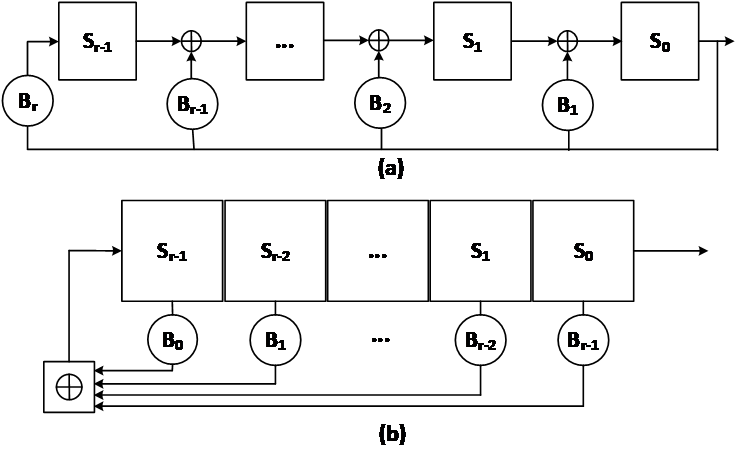### 2. Implemented LFSR-based Shrink Generator

Although LFSRs are good sources of random numbers, maximal-length LFSR sequences can be entirely reconstructed using the Berlekamp-Massey algorithm when only 2N consecutive output sequences are known. This renders the traditional LFSR linearly simple. The Shrink Generator (SG) proposed by  improved on the linear complexity of LFSRs to make the output sequence secured and resistive to attacks. This proposed architecture used two maximal-length LFSR’s pseudorandom bits to generate a third pseudorandom bit that is of better and higher statistical quality than the initial two used in generating it. Assuming the first LFSR, A$_{1}$, constructed from the primitive polynomial p$_{1}$(x), is of length T$_{1}$and the second LFSR, A$_{2}$, constructed from the primitive polynomial p$_{2}$(x) is of length T$_{2}$, where T$_{1}$< T$_{2}$. If the set of PN-sequences generated by A$_{1}$ and A$_{2}$ are {j$_{0}$, j$_{1}$, j$_{2\ldots }$} and {k$_{0}$, k$_{1}$, k$_{2}$…} respectively, then the sequence {j$_{0}$, j$_{1}$, j$_{2\ldots }$} decimates the sequence {k$_{0}$, k$_{1}$, k$_{2}$…} to result in $\left\{ss_{0},ss_{1},ss_{2}\ldots \right\}$, which is referred to as the shrunken sequence, using the decimation rule in Eq. (1).

##### (1)
$\left\{ss_{0},ss_{1},ss_{2}\ldots \right\}=\left\{\begin{array}{l} k_{i},\,\,\,\,\,\,\,\,\,\,\,\,\,\,\,\,\,\,\,\,if\,\,j_{i}=1\\ discard\,\,k_{i},\,\,if\,\,j_{i}=0 \end{array}\right.$

The primitive polynomials used for LFSRs A$_{1}$$_{{,}}$ and A$_{2}$, are shown in Eqs. (2) and (3) respectively. Fig. 3 shows the architecture of the implemented design. A maximal-length LFSRs that generate about 120-bit pseudorandom sequences were used. The module takes in as input a clock signal clk, an rst signal for system reset, and an init signal to initialize the LFSRs to an initial state using the seed value. To increase the level of security, the selector LFSR, (A$_{1}$), uses the mask of the primitive polynomials as its seed or initial state. Finally, an enable signal, en, is used to toggle the states of the LFSRs. After each clock cycle, the bit sequences from LFSRs A$_{1}$ and A$_{2}$ are fed to the block named Shrinker in Fig. 3. The shrinker implements the decimation logic stated in Eq. (1).

##### (2)
$x^{122}+x^{121}+x^{120}+x^{116}+1$
##### (3)
$x^{120}+x^{118}+x^{114}+x^{111}+1$

It is a combinational circuit based on the generate for-loop to roll out the individual bits of the sequence and perform the decimation during logic synthesis. A temporary register, the size of the LFSR A$_{{2}}$, is used to temporarily hold the decimated value. The period of a Shrink Generator can be determined or computed from the individual periods of the two LFSRs employed in the design of the shrink generator. Eq. (4) and  show the expression required to compute the period. It must be noted that this expression assumes the greatest common divisor of the periods of the polynomials is 1.

##### (4)
$period\left(SG\right)=2^{{T_{1}}-1}\left(2^{{T_{2}}}-1\right)$

To confirm the operation of the designed SG, 20000 samples were captured from the generated sequences. The uniform distribution of the sequences shown in Fig. 4 confirms the operation of the SG’s implementation. The irregularly decimated PN-sequences possess good cryptographic qualities including longer periods with better correlation, and easy implementation both in hardware and software. For these advantages, we opted to implement the shrinking generator among the family of irregularly decimated PN-sequence generators. Sequences generated from the SG were used as intermediary challenges of a CRO-PUF to generate PUF responses of high statistical quality. The SG output sequences are not used directly as done in the case of some stream ciphers hence they did not pose any statistical disadvantage for this research.

##### Fig. 3. Implemented shrink generator architecture.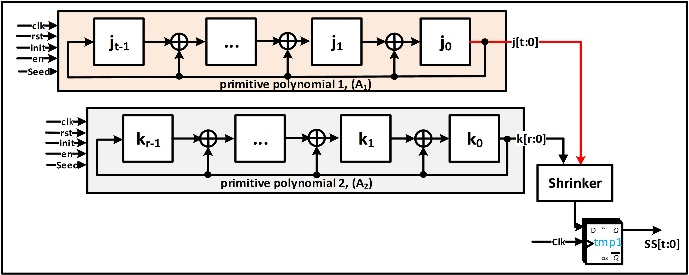##### Fig. 4. Uniform distribution graph of shrink generator sequences.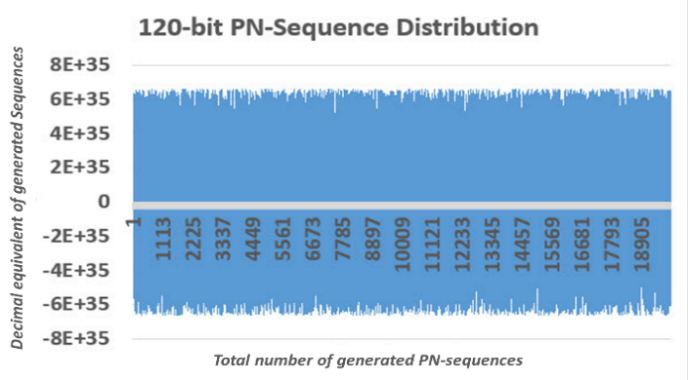## IV. CONFIGURABLE RING OSCILLATOR (CRO) PUFS

### 1. Related Works on CRO-PUFs

Delay-based silicon PUF architecture was first introduced by . The ring oscillator, one of the earliest and most matured among this class of PUF introduced in , has its architecture made up of several ring oscillators. In the year 2012, the Anderson PUF —the first PUF design to be implemented on an FPGA—was proposed. From this time onwards, the major drawback of the past and current forms of RO-PUF is their reliability.

The RO-PUF possesses considerable levels of instability among its response bits when variations in environmental and operating conditions exist. For this reason, several RO-PUFs require some form of error-correcting code such as BCH, to improve upon its reliability. In a bid to enhance the stability or reliability of the bits, several techniques have been proposed within the past years through research to help improve upon the reliability of PUFs to eliminate the use of error-correcting codes. The 1-out-of-N (C)RO-PUF in Fig. 5 was proposed. However, the architecture meant that a single response bit rendered (n-2) unused ROs as a hardware overhead. Not all, Cao et al.  in their study presented on the use of the negative temperature coefficient of an inverter deprived of current to maintain a balance within the positive coefficient of the regular RO. This improved the reliability against the temperature variations. This proposed architecture equally incurred hardware overhead as (n-1) PUF units were employed to generate a single bit. Additionally, the use of reconfigurability shown in Fig. 6(a) was introduced by  as a way of improving reliability. This design relied on 2-to-1 multiplexers to select one out of two inverter gates at each stage and used the configuration with the largest difference in the delay to improve upon reliability. A similar architecture of high flexibility in Fig. 6(b), proposed by  relied on a 2-to-1 multiplexer to either include or exclude the inverter in a stage of the oscillator chain. For these proposed CRO-PUFs architectures, the ratio of multiplexer inclusion to its usage was low whereas the inverters are also not fully utilized in some configurations. Although these architectures are very suitable for FPGA implementation, there exists a lot of hardware overhead and low efficiencies when used.

This research, therefore, proposes a configurable RO-PUF architecture with properties of a Strong-PUF, with high reliability among the response bits. A comprehensive discussion of the proposed PUF architecture, as well as the statistical analysis to ascertain the level of functionality and quality of response bits obtained, are presented in the following sections.

##### Fig. 5. Traditional 1-out-of-N ring oscillator PUF architecture.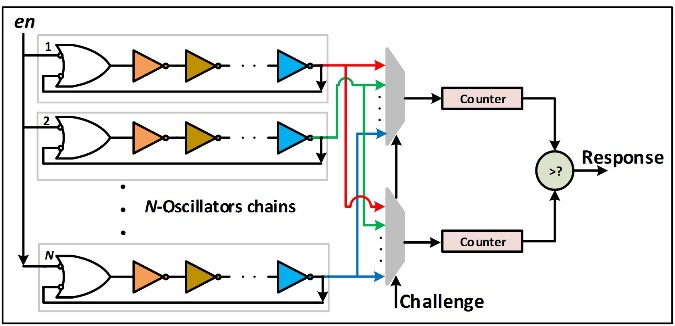##### Fig. 6. (a) CRO-PUF; (b) CRO-PUF; (c) Proposed CRO-PUF.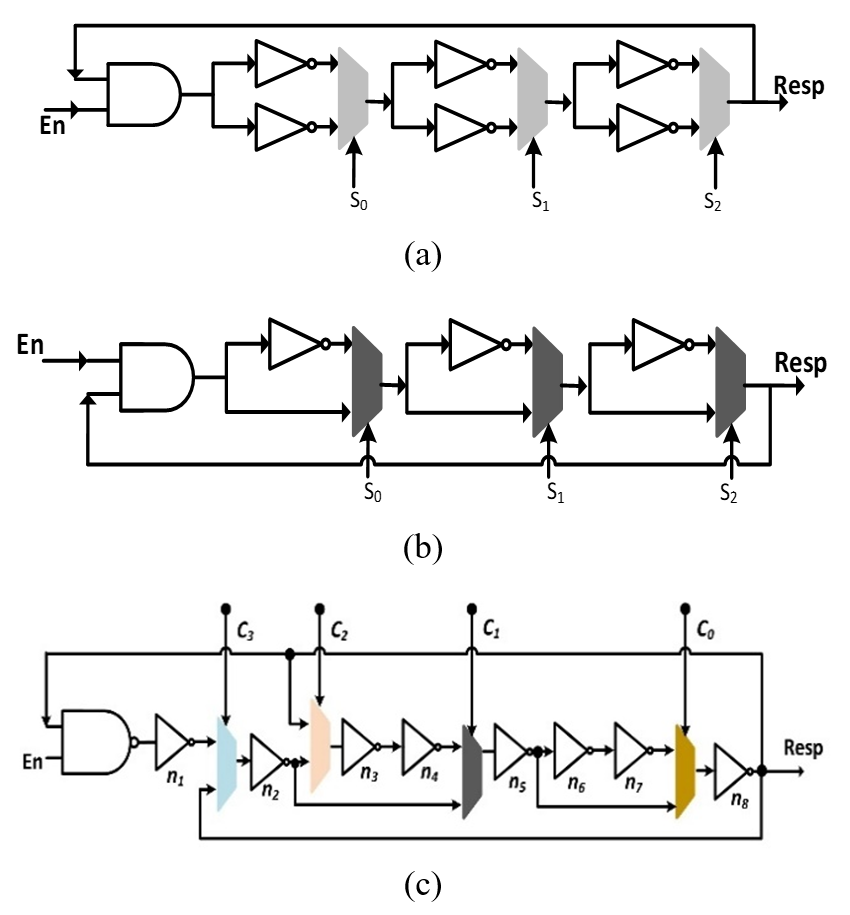### 2. Proposed CRO-PUF Architecture

The proposed architecture was inspired by the proposed designs of Maiti et al  and Gao et al . It can be noticed that the input to an inverter can be obtained at the output when an even number of inverters are used. To introduce configurability and increase the stability of the oscillator rings which translates to a degree of reliability in the response bits, it was observed that virtually creating a 2-input NOT gate'' proved effective at ensuring that the same signal level is available at a NOT gate. Since every logic gate and wires individually have propagation delays, the signal that is one stage before and the signal that is 3 stages away from the current stage can be fed into a 2-to-1 multiplexer as shown in Fig. 6(c). This setup ensures the same signal level is always present. The presence of the same signal level means that the oscillating frequencies do not fluctuate intermittently but produce a smooth and continuous sign wave verified in Fig. 7. Additionally, the propagation delays of the different paths provide the needed advantage of configurability. Generally, an n-th stage inverter can be driven by the outputs of either n-1 or n-3 inverters through a multiplexer. This proposed architecture replaces the two cascaded rows of inverters architecture in Fig. 6(a) with a single row of inverters.

The proposed approach also maintains the same signal level at an inverter’s input and thus, does not distort the RO property but rather introduces configurability. For this architecture, each inverter stage of the RO does not necessarily require a multiplexer. The minimum number of multiplexers required for a design can be determined based on the statistical examination of the responses. If N-number of multiplexers are used, then a total of $2^{N}$possible configurations can be realized. The variations in the oscillating frequency based on the select signals of the multiplexer can be calculated from Eq. (5), where $n_{s}~$ is the number of inverters and $t$, the propagation delay of the selected signals. Fig. 7 and Table 1 randomly show the varying frequencies based on the multiplexers’ select signals (challenge bits) combination for an oscillator chain.

##### (5)
$Freq_{CRO}=\frac{1}{2n_{s}t}$
##### Fig. 7. The oscillating frequency of the proposed PUF architecture with (a) 1111; (b) 1110 multiplexer select bits sequences.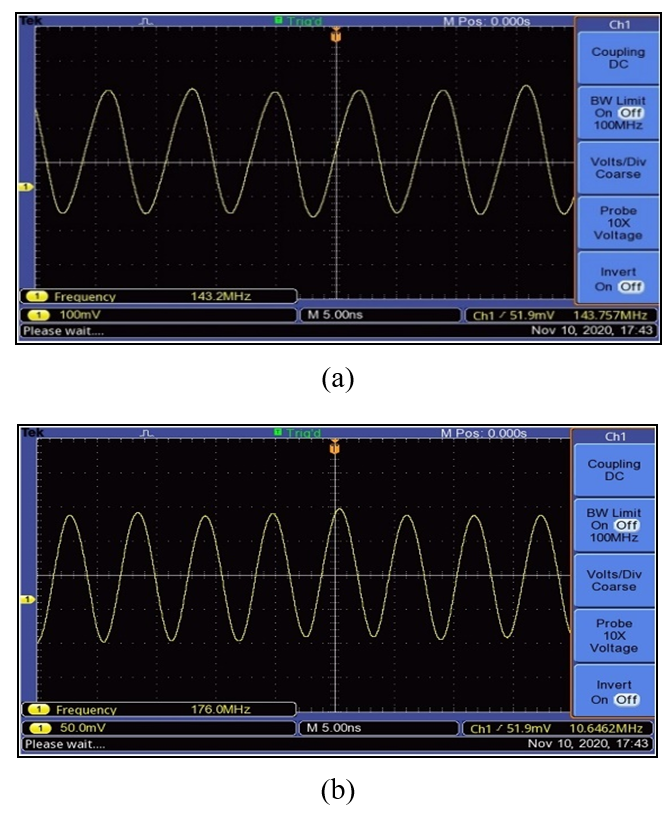##### Table 1. challenge selection bits with their corresponding oscillating frequency
 Challenge bits Frequency of Oscillation (MHz) 4’b0000 128.2 4’b0010 115.4 4’b1001 189.6 4’b1010 98.63

## V. RESULTS AND DISCUSSION

### 1. Experimental Setup

The experimental setup consists of two main components—an FPGA chip and a Personal Computer. As several chips are required in testing a PUF architecture, a total of 6 Spartan-6 FPGA chips fitted on the Combo II-DLD board were used in evaluating the proposed design. Fig. 8 shows the block diagram of the experimental setup for the evaluation. The module that is implemented on the FPGA chip has four submodules. The main submodule is the proposed composite PUF architecture shown in Fig. 9. This consists of a set of 120 ring oscillator chains labeled CRO$_{0}$ to CRO$_{n-1}$. Each Identical CRO chain has 8 inverter gates, 4 2-to-1 multiplexer gates, and a NAND gate. At the sampling end of each CRO chain, a D-latch is used to sample the response bits. The proposed architecture also consists of a Shrink Generator (SG LFSR) core, discussed in Section III, that generates PR sequences used as intermediary challenges for the CROs. A controller block—en-gen controller—controls and monitors the proposed architecture's operation. Additionally, the proposed architecture also consists of three vectors labeled T1, T2, and T3. These vectors are used for voting purposes that result in a temporary response vector V1 after the voting. A fifth vector—V2—is an additionally generated PR sequence vector from the SG LFSR core. Other blocks in the experimental setup are the MicroBlaze MCS soft processor core. This core allows for the writing of commands to and dumping of generated response data from the FPGA chip on which the PUF architecture has been implemented, to the Personal Computer through the UART communication protocol for data analysis. A memory block that is generated using Xilinx’s IP CORE generator holds the challenge and response bits. The memory blocks are 120-bits wide and 1024 deep. These memory blocks hold randomly generated 120-bits from a software application that are used as initial challenges or seeds. The FSM Controller is the final submodule of the FPGA implemented experimental module. This submodule, which is mainly an FSM, schedules the interoperability of all the blocks in the experimental setup.

At the start of an operation to generate PUF responses, the shrink generator (SG LFSR) is first initialized and enabled by the init and lfsr_en signals. A challenge is read from the ROM and applied to SG LFSR as a seed. After all the inputs signals are provided, the SG LFSR which is controlled by an internal state machine generates 600 bits long of PR sequences. Out of these, 480 bits are used as the intermediary challenge bits—$C_{1},C_{2},\ldots ,C_{4n-1}$—of the 120 CRO chains, 4-bits per oscillator chair as shown in Fig. 6(c). The remaining 120 bits of the earlier generated PR sequence are kept as vector V2 for use during the latter stages of response generation. After the completion of the SG-LFSR’s operation, which is indicated by a done signal, the en-gen controller block generates a cro_en signal that triggers the oscillation of the CRO chains. To increase the degree of measurement accuracy of the response bits, the CRO chains are allowed an average warmup cycle. This is done by taping the output of one oscillator chain and monitoring it for a specified threshold warmup cycle. A threshold of 253 was set as the warmup cycle for this experiment.

At the realization of the warmup threshold, a D-latch_en signal is generated by the en_gen controller to latch the outputs of all the CRO’s. The D-latch_en signal is de-asserted on the next threshold count—count 254. This is then followed by the de-assertion of the cro_en signal to completely stop the oscillation and reset the warmup threshold counter. To further reduce the noise in the response bits and increase reliability a simple majority voting scheme was implemented. For this purpose, CRO enabling, warmup monitoring, and response latching cycles are repeated 3 times in total. After each generation, the latched responses are kept in one of the three sampling vectors labeled T1, T2, and T3. These three vector samples are passed through a majority voting scheme  which operates by checking the frequently occurring response bit within the three vector samples T1, T2, and T3 to obtain the responses in the V1 vector. It must be noted that, for a majority voting scheme, an odd number of voters—response bits in this case—is desired. Based on the analysis, which is explained further in the subsection that follows, there is some likelihood—very small—of an unbalanced number of 1’s and 0’s in V1 to occur. Owing to this reason, the responses might fail a frequency statistical test. However, since an XOR operation of a random and non-random vector results in a random vector. Based on this fact, randomness is introduced into the partial responses in vector V1 by an XOR operation with vector V2 to obtain the final CRO-PUF output Response (Resp.) bits.

##### Fig. 8. Experimental setup of the proposed architecture.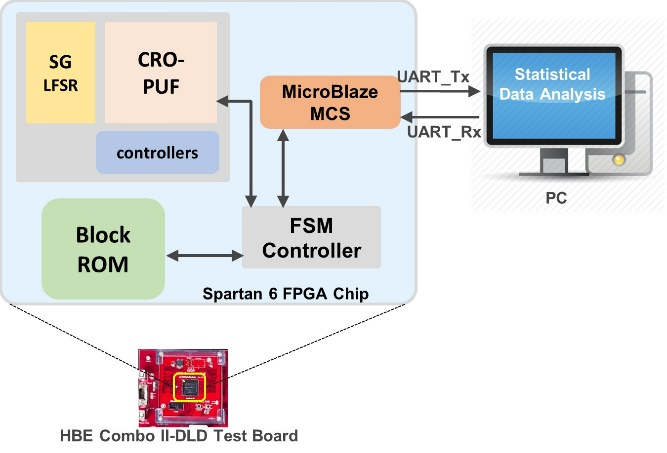##### Fig. 9. Proposed PUF architecture.### 2. Statistical Analysis of Results

A PUF’s responses are generally considered random variables having characteristic probability distribution which are usually determined from hardware experiments or simulations. Three vital statistical measures are observed within the response bits of a PUF to mathematically determine the suitability of a PUF architecture’s usage. These statistical measures are randomness, uniqueness, reliability analysis to which the proposed PUF architecture’s responses are subjected, analyzed, and results presented.

#### A. Randomness

The randomness statistical test component analyzes the responses of a PUF to determine the distribution ratio of 1’s and 0’s. This randomness value, which also reflects the uniformity, should have an Ideal value of 50%. Therefore, the number of 1’s and 0’s must be equally probable in any sampled response. To easily perform this test, the NIST statistical test suite  was used. To establish the uniqueness of the proposed design, the same PUF architecture was initially tested without the use of SG LFSR. In this setup, all the challenge bits were tied to ground. The response bits from the PUF were measured 1024 times and on 6 different FPGA chips. The resulting PUF responses were then passed through the statistical test suite by NIST.

The results for 8 of the 15 available statistical tests in the NIST suite are shown in Fig. 10(a). It was observed that the response bits failed the statistical test. This failure is associated with the closely similar oscillating frequencies of the neighboring ring oscillator chains. For this reason, the bits in the response had continuous runs of 1’s or 0’s for the individual response of 120 bits. Not all, there was also an uneven distribution of 1’s and 0’s in the response. For each 120-bit response, an average of 65 0’s and 35 1’s was recorded. On a larger scale, the total number of 1’s in the response bits was 44,463 out of the 122,880 total bits generated which represent 36.18% of the 1’s and the remaining 63.81% representing the 0’s. Similar results were also obtained from the remaining 5 FPGA chips.

The test for the architecture that includes the SG LFSR to generate the configurability bits was performed next. Similarly, a total of 1024 by 120-bits by 6 FPGAs equaling 80 KiB of data was generated and passed through the test suite. It must be noted that the data set generated was passed through the simple voting scheme and not XORed with the V2 vector. Significant improvement of 48.37% of 1’s and 51.63% of 0’s was observed using the voting scheme and the configurable bits. This shows the desirable effect of the configurable bits on the sampled PUF responses. From Fig. 10(b) it can be noticed that the responses passed most of the test while failing a few with good proportions. Finally, in the randomness test, the responses are generated where vector V2 is used to introduce randomness into the V1 vector was performed and the results are shown in Fig. 10(c). This was done in the order to reduce to the minimum, if not eliminate the likelihood of any failure such as that recorded in Fig. 10(b). From Fig. 10(c), the minimum pass rate is indicated as 96 for a sample size of 100 and a P-value greater than $\alpha =\left(0.01\right).$ This result is associated with the configurable bit’s influence on altering the operating frequencies of even neighboring RO as indicated in Fig. 6(c).

##### Fig. 10. NIST statistical test report: (a) using no configurable bits; (b) using configurable bits; (c) using configurable bits with response bits and vector V2 XORed.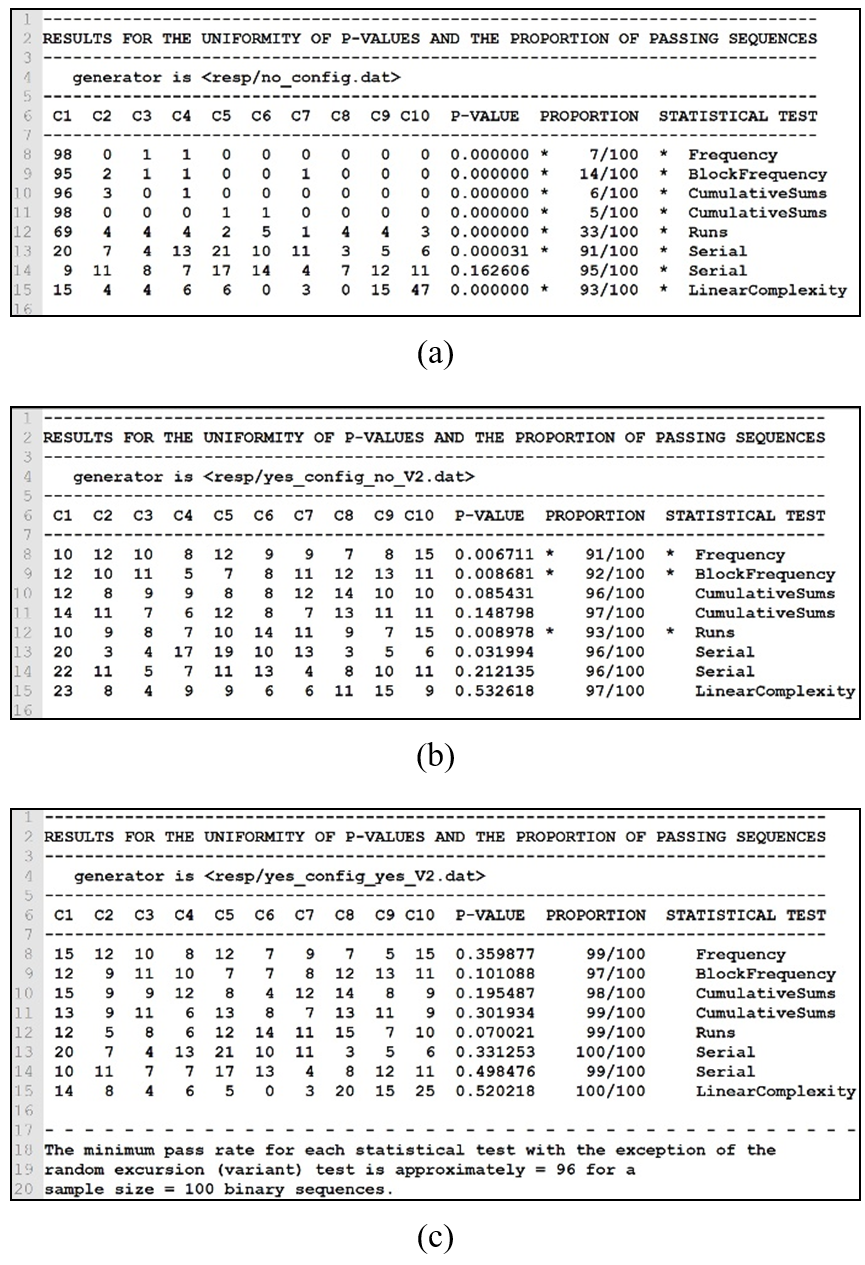#### B. Uniqueness

A PUF’s uniqueness refers to the analysis of responses from different PUF instances or chips. Inter hamming distance $HD_{inter}$ therefore measures the differences in the responses of PUF instances or chips evaluated with the identical set of challenges and drawn two at a time. This measure hence expresses how unique the responses of single PUF architecture are for every implementation instance or chip. The Ideal value for Inter-chip Hamming Distance ($HD_{inter}$) which also reflects the uniqueness is 50%. This inter-chip variation is computed using the defined in Eq. (6).

##### (6)
$HD_{inter}=\frac{2}{m\left(m+1\right)}\sum _{i=1}^{m-1}\sum _{j=i+1}^{m}\frac{HD\left(R_{i},R_{j}\right)}{n}x100$

where $R_{i}$ and $R_{j}$ are the n-bit responses generated from two PUF instances $i~$and $j$ using the same challenge and $m~$represents the total number of chips or instances tested. To test the uniqueness of the proposed PUF architecture, each chip was tested with 1024 x 120-bit challenges, and a total of 1024 x 120-bit = 122880-bit responses were sampled per PUF instance or Chip. The $HD\left(R_{i},\,\,R_{j}\right)$ were computed for all the possible combinations of the 6 FPGA test chips. This implies that $\mathrm{\mathbb{C}}\left(6,2\right)=15$ possible HD values were computed. Using these obtained $HD_{inter}$ values, the histogram distribution in Fig. 11 was derived.

##### (7)
$Std_{binomial}=\sqrt{s~ x~ p~ x~ \left(1-p\right)}$

The Ideal value for uniqueness is 50% therefore, for our data size of 122,880 bits, the ideal average should be 61,440 bits. Since the distribution is binomial, the standard deviation can also be computed using Eq. (7) where the variables $s,$ $p,$ and $1-p$ represent the total of bits and the probability of occurrence of 1 and 0 respectively. By computation, the ideal standard deviation should also 175.3. Comparing this to the Histogram plotted Fig. 11, the actual mean $HD_{inter}$ is 61,457. This represents 50.01% of the difference in responses and is very close to the ideal average of 61440. Similarly, from Table 2, the actual standard deviation from our experiment is 172.38 which is also close to the Ideal figure of 175.3. The minimum and maximum average inter-hamming distance as well as the sum of all the 15 comparisons are also indicated in Table 2. These analyses, therefore, show that the proposed PUF architecture generates unique responses for different instances or chips.

##### Fig. 11. Histogram of inter-chip hamming distance.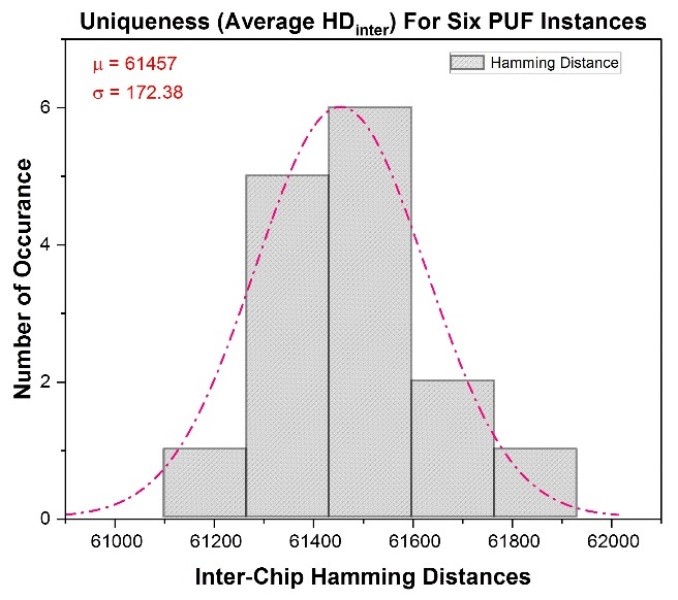##### Table 2. Statistical summary on the 15 averages $HD_{inter}$ from the 6 FPGA chips
 $\mu$ $\sigma$ Sum Min. Med. Max. 61457 172.383 921855 61203 61436 61842

#### C. Reliability

Reliability deals with the analysis of responses from the same PUF instance or chip, using the same set of challenges but under different environmental conditions such as temperature and voltage. This test determines the noise margins within the responses when variations in environmental conditions such as temperature and/or voltage are applied. This quality measure is determined by the distribution of the intra-hamming distance $HD_{intra}$. The mathematical expression to compute the $HD_{inter}$ is shown in Eq. (8) where $R_{i}$ represents an n-bit response generated at the ideal operating conditions—25$\mathrm{℃}$ and 3.3 V—of the FPGA chips, in the case of the FPGAs used in this research. $R_{i,j}$ represents the responses obtained when the same challenge bit is applied $K$ times under variable environmental conditions.

##### (8)
$HD_{intra}=\frac{1}{K}\sum _{j=1}^{K}\frac{HD\left(R_{i},R_{i,j}\right)}{n}x100$

To evaluate the reliability, four Temperature-Voltage (TV) corners were defined. These corner conditions set for the reliability test are as follows:

1. Low temperature-Low voltage, LL, (5$\mathrm{℃}$, 1.8 V)

2. Low temperature-High voltage, LH, (5$\mathrm{℃}$, 4.8 V)

3. High temperature-Low voltage, HL, (50$\mathrm{℃}$, 1.8 V)

4. High temperature-High voltage, HH, (50$\mathrm{℃}$, 4.8 V)

The reference response bits, which are sampled at the enrollment phase, measures the response bits at the optimal TV conditions of the FPGA. A total of 1024 x 120 bits were sampled during enrollment. The next phase which is the regeneration phase was performed next. Using the same set of challenges in the enrollment phase, response bits for each of the TV corners were regenerated 10 times per PUF instance. Therefore, for a TV corner, a total of 1024 x 120-bits x 10 regenerations were measured. The enrollment response bits $R_{i}$ and the regenerated response bits $R_{i,j}$ where $j=\left\{1,2,\ldots 10\right\}$ are used to compute the Hamming distances for each set of regenerated bits. The 10 hamming distances are computed for each TV corner to obtain an $HD_{intra}$ value. The average of the $HD_{intra}$ from all the other TV corners are then computed to obtain the average $HD_{intra}$ for the PUF instance. Fig. 12 shows a line graph of the individual TV corners measured. The average $HD_{intra}$ for an FPGA was 3.562 which is not the Ideal 0 required but tolerable for certain applications. Using Eq. (9), the reliability of the proposed PUF on FPGA 1 was computed to be $\left(100-3.562\right)=96.43\,\,\mathrm{\% }$.

##### (9)
$Reliability=100-HD_{intra}$

The 1-out-of-N ring oscillator PUF’s are usually reliable because they eliminate, to a certain degree, most of the noise that may be present in a set of response bits by comparing the frequencies to obtain a single response bit. However, in this experiment, since the frequencies on the CRO chains were not compared to obtain a single response bit, the high rates of the reliability observed for the proposed PUF architecture are attributed to the threshold frequency monitoring and voting scheme approach. The warmup approach allowed the oscillators to oscillate for a specified number of oscillations to gain stability before the outputs are sampled whereas the voting scheme that was implemented also enhanced reliability by filtering out some of the noisy bits that translates into the reliability level of the PUF architecture. A summary of the average of 10 $HD_{intra}$ for each TV corner condition is and the overall average for one FPGA is shown in Table 3.

##### Fig. 12. Reliability test on varying voltages and temperatures.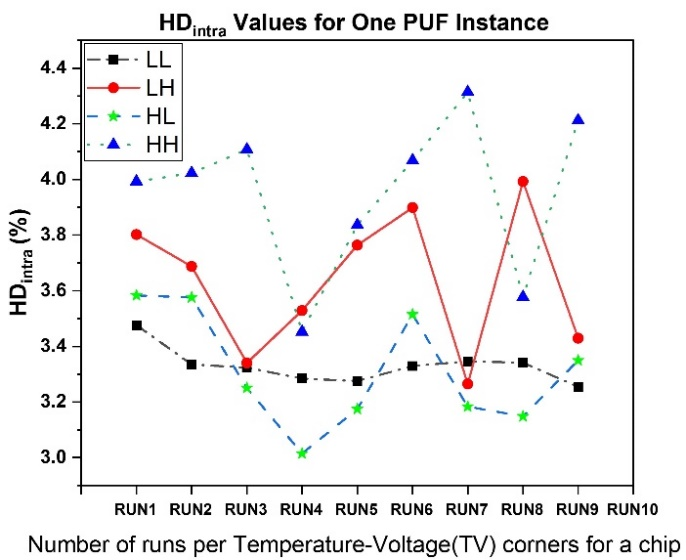##### Table 3. Summary of the average intra-hamming distances for the temperature-voltage corners for a PUF instance on an FPGA
 LL LH HL HH Avg. $HD_{intra}$ 3.322 3.626 3.304 3.998 3.562

#### D. Results Comparison

Table 4 summarizes a comparison of the statistical measures and resource utilization of some existing Strong and Weak architectures. From the reported results, the proposed PUF architecture demonstrates desirable uniqueness of 50.01% for a traditionally Weak PUF. For reliability, the proposed architecture demonstrates to be competitive to architectures in [4,10,20] being 96.4% reliable. The Chip Scope Pro internal logic analyzer in Fig. 13 shows the captured accuracy of multiple regenerations of responses from the proposed PUF architecture in operation on an FPGA. From Eq. (4), the Challenge space of the shrink generator, based on the primitive polynomials 1 and 2 in Eqs. (2) and (3) is $8.834x10^{71}$.

Considering the SG LFSR is run 16 times to generate the set of 420 multiplexer signals and an additional 4 times to generate the random vector V2, the period for the Shrink generator reduces to $4.417x10^{70}$. Comparing this to a traditional 120-stage LFSR’s period of $1.329x10^{36}$, there is an approximately 100% increment in the CPR set. Based on this, the proposed CRO-PUF qualifies as a Strong PUF choice for applications that require larger CPR sets with increased reliability and uniqueness.

##### Fig. 13. Xilinx’s Internal Logic Analyzer showing proposed PUF’s signals: (a) start trigger; (b) shrink generator enable and done signals for generating the internal multiplexer select vector and the V2; (c) CRO-PUF block ready strobe signal to register values for V1; (d) strobe signal indicating completion of generation the availability of the response.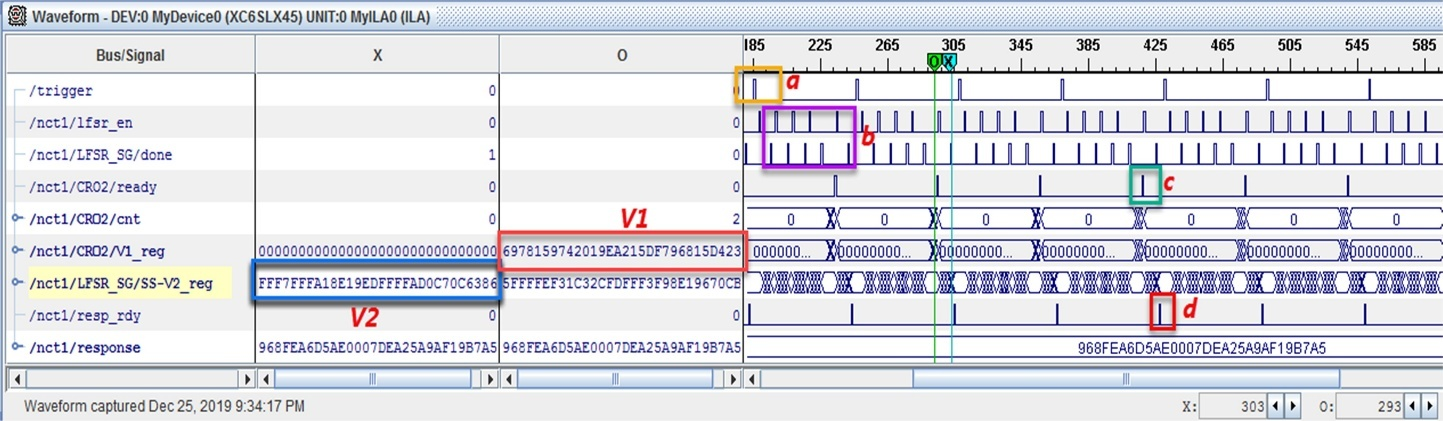##### Table 4. Hardware resource utilization, uniqueness, reliability, and response length result comparison
 PUF Design U (%) R (%) L Device SLICES Arbiter PUF  29.2 99.2 64 Spartan 6 - Feedforward Arbiter PUF  38 90.2 - TSMC 0.18 µm - CRO-PUF  43.5 96 128 Spartan 3 7 x 128 L / LR-PUF  50.25/ 49.66 96.3 64 Artix-7 210 / 129 This Work 50.01 96.4 120 Spartan 6 780
U = uniqueness, R = Reliability, L= Length of response

## VI. CONCLUSION AND FUTURE WORK

The realization of a Strong PUF that is based on a Shrink Generator and a Weak CRO PUF has been proposed in this paper. The option to use a shrink generator was due to its larger period compared to the ordinary LFSR. As a result, the PR sequences realized serve as an exponential source of challenge-response pairs. Not all, multiplexers were used to introduce configurability into the classic ring oscillator by positioning them such that there is always the same logic level signal present at the input of the NOT gate. This layout causes the NOT gate, to which the mux is attached, to operate as though it were a 2-input, 1-output'' gate which enhances the stability of the oscillating ring. The inclusion of the multiplexers in this configuration allows the ring oscillator to be configured in a total of $2^{n}$ possible ways leading to an increase in both uniqueness and reliability of the proposed architecture. Since the raw challenges are not applied directly to the CRO-PUF, an inherent level of security is introduced in the proposed PUF architecture. Traditional RO-PUFs are highly suitable for FPGA designs however, they lack the required number of CRPs that allow their use in both identification and authentication applications. As a solution, the proposed architecture provides the needed exponential CRPs. The hardware area and the simplicity of the proposed PUF architecture make it suitable for resource-constrained devices. Experimental results compared to that of other modern CRO-PUFs architectures indicate that the proposed PUF passes the NIST randomness test. It also has its reliability and uniqueness to be 96.43% and 50.01%, respectively. This, to a degree, confirms the proposed architecture’s suitability for use as either a Weak or Strong PUF but may require further error-correcting schemes. For future work, a larger data set will be obtained by increasing the number of test chips which will allow for further statistical and security analysis such as the modeling attack resistance evaluation. The resulting architecture will be integrated into an SoC-based elliptic curve integrated encryption scheme architecture to serve as a source of private keys.

## References

1
Apple iPhone 7 Teardown. Accessed: May 21, 2019. [Online]. Available: https://www.techinsights.com/blog/apple-iphone-7-teardown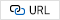2
A. Tilley, “This Mysterious Chip In the iPhone 7 could be key to Apple’s AI Push”, Available: https://www.forbes.com/sites/aarontilley/2016/10/17/iphone-7-fpga-chip-artificial-intelligence/#77e2d3ca3c693
S. McNeil, “Solving today’s design security concerns”, Xilinx, White Paper WP365(v1.2), pp. 14, 2012.4
A. Maiti, P. Schaumont, "Improved Ring oscillator PUF: An FPGA-friendly secure primitive," Journal of. Cryptology, pp. 375-397, Oct. 2010.5
G. E. Suh, S. Devadas, "Physical unclonable functions for device authentication and secret key generation" In Proceeding of 44th ACM/IEEE Design Automation Conference, pp. 9-14, Jun. 2007.6
R. Pappu, B. Recht, J. Taylor, N. Gershen-Feld, "Physical one-way functions", Science, vol. 297, pp. 2026-2030, Sept. 2002.7
H. Handschuh, G. J. Schrijen, and P. Tuyls, “Hardware intrinsic security from physically unclonable functions,” Towards Hardware-Intrinsic Security, pp. 39-53, Oct. 2010.8
D. Merli, F. Stumpf, and C. Eckert, “Improving the quality of ring oscillator PUFs on FPGAs,” Embedded Systems Security, 5th ACM Workshop on, p. 9, Oct. 2010.9
M. Gao, K. Lai, and G. Qu, “A highly flexible ring oscillator PUF,” in 2014 Design Automation, 51st ACM/EDAC/IEEE Conference on, pp. 1-6, Jun. 2014.10
Y. Hori, H. Kang, T. Katashita, A. Satoh, S. Kawamura, and K. Kobara, ‘‘Evaluation of physical unclonable functions for 28-nm process field-programmable gate arrays,’’ Journal of Information Processing, Vol. 22, No. 2, pp. 344-356, Apr. 2014.11
D. Lim, et al, "Extracting secret keys from integrated circuits," Very Large Scale Integration. (VLSI) Systems, IEEE Transactions on, Vol. 13, No. 10, pp. 1200-1205, Dec. 2005.12
Texas Instruments Incorporated, “What’s an LFSR?”, SCTA036A, Dec. 1996.13
R. Vara Prasad Rao, N. Anjaneya Varaprasad, G. Sudhakar Babu, and C. Murali Mohan, "Power Optimization of Linear Feedback Shift Register (LFSR) for Low Power BIST implemented in HDL", International Journal of Modern Engineering Research (IJMER), Vol. 3, pp. 1523-1528, May 2013.14
D. Coppersmith, H. Krawczyk, and Y. Mansour “The Shrinking Generator” Advances in Cryptology — CRYPTO’ 93, CRYPTO 1993, Lecture Notes in Computer Science, Vol. 773, pp. 22-39, 1994.15
S. Cardell, A. Fúster-Sabater, and A. Ranea, “Linearity in decimation-based generators: an improved cryptanalysis on the shrinking generator,” Open Mathematics, Vol. 16, No. 1, pp. 646-655, Jun. 2018.16
A. Mills, S. Vyas, M. Patterson, C. Sabotta, P. Jones and J. Zambreno, “Design and evaluation of a delay-based FPGA physically unclonable function,” Computer Design, 30th IEEE International Conference on, pp. 143-146, Oct. 2012.17
Y. Cao, L. Zhang, C.-H. Chang, and S. Chen, “A Low-Power Hybrid RO PUF with Improved Thermal Stability for Lightweight Applications,” Computer-Aided Design of Integrated Circuits Systems, IEEE Transactions on, Vol. 34, No. 7, pp. 1143-1147, Jul. 2015.18
J. Ye, Y. Hu, X. Li, “VPUF: Voter based Physical Unclonable Function with High Reliability and Modeling Attack Resistance,” International On-Line Testing Symposium (IOLTS), 23rd IEEE Conference on, pp. 74-79, Jul. 2017.19
E. Lawrence, L.E. Bassham III, et al., “SP 80022 rev. 1a. a statistical test suite for random and pseudorandom number generators for cryptographic applications,” National Institute of Standards and Technology (NIST), Apr., 2010.20
S. Hou, Y. Guo and S. Li, "A Lightweight LFSR-Based Strong Physical Unclonable Function Design on FPGA,” Access, IEEE, Vol. 7, pp. 64778-64787, May 2019.Guard Kanda was awarded a BSc. Degree in Computer Science from Kwame Nkrumah University of Science and Technology, Ghana, in 2012 and an M.Eng. Degree in Information and Communication Engineering from Hanbat National University, South Korea in 2016. In 2017, he began his Ph.D. research on the topic of hardware cryptographic architectures in the same department and graduated in 2022. His research interests include SoC Design and Verification Platforms, Lightweight Cryptography, Hardware, and Embedded Systems Security, and Hardware-Software co-design.

Kwangki Ryoo received the BSc., MSc, and Ph.D. Degrees in Elec-tronic Engineering from Hanyang University, Korea in 1986, 1988, and 2000 respectively. From 1991 to 1994, he was an Assistant Professor at the Korea Military Academy (KMA) in South Korea. He later worked as a Senior Researcher in IC Design Department at the Electronics and Telecommunications Research Institute (ETRI), Korea, from 2000 to 2002. He was a visiting scholar at the University of Texas in Dallas from 2010 to 2011. Since 2003, he has been a Professor at Hanbat National University, Daejeon, Korea. His research interests include Engineering Education, SoC Platform Design and Verification, Image Signal Processing and Multimedia Codec Design, and SoC Design for Security.